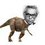# RMO Inequality practice (2) (Original)

If $$a,b,c$$ are positive reals and $$n$$ is a positive integer , Prove the following inequality:

$\large\dfrac{na}{b+nc} + \dfrac{nb}{c+na} +\dfrac{nc}{a+nb} \geq \dfrac{3n}{n+1}$

###### This problem is original and is inspired by some note.Note by Nihar Mahajan
5 years, 2 months ago

This discussion board is a place to discuss our Daily Challenges and the math and science related to those challenges. Explanations are more than just a solution — they should explain the steps and thinking strategies that you used to obtain the solution. Comments should further the discussion of math and science.

When posting on Brilliant:

• Use the emojis to react to an explanation, whether you're congratulating a job well done , or just really confused .
• Ask specific questions about the challenge or the steps in somebody's explanation. Well-posed questions can add a lot to the discussion, but posting "I don't understand!" doesn't help anyone.
• Try to contribute something new to the discussion, whether it is an extension, generalization or other idea related to the challenge.

MarkdownAppears as
*italics* or _italics_ italics
**bold** or __bold__ bold
- bulleted- list
• bulleted
• list
1. numbered2. list
1. numbered
2. list
Note: you must add a full line of space before and after lists for them to show up correctly
paragraph 1paragraph 2

paragraph 1

paragraph 2

[example link](https://brilliant.org)example link
> This is a quote
This is a quote
    # I indented these lines
# 4 spaces, and now they show
# up as a code block.

print "hello world"
# I indented these lines
# 4 spaces, and now they show
# up as a code block.

print "hello world"
MathAppears as
Remember to wrap math in $$ ... $$ or $ ... $ to ensure proper formatting.
2 \times 3 $2 \times 3$
2^{34} $2^{34}$
a_{i-1} $a_{i-1}$
\frac{2}{3} $\frac{2}{3}$
\sqrt{2} $\sqrt{2}$
\sum_{i=1}^3 $\sum_{i=1}^3$
\sin \theta $\sin \theta$
\boxed{123} $\boxed{123}$

Sort by:

Prove ${\frac{a^3}{a^2+ab+b^2}+\frac{b^3}{b^2+bc+c^2}+\frac{c^3}{c^2+ac+a^2}}\geq{\frac{3abc}{ab+bc+ca}}$

- 5 years, 2 months ago

$a,b,c$ are positive reals right?

- 5 years, 2 months ago

Yes. It is a nice problem :) But there is a stronger one after.

- 5 years, 2 months ago

I got inspired by this problem. I am posting something (its easy though). Stay tuned :P

- 5 years, 2 months ago

:) Nice.

- 5 years, 2 months ago

- 5 years, 2 months ago

$\large {\sum_{cyc} \dfrac{a^3}{a^2+ab+b^2} \\ = \sum_{cyc} \dfrac{a^4}{a(a^2+ab+b^2)} \\ \geq \dfrac{\left(\displaystyle\sum_{cyc} a^2\right)}{\displaystyle\sum_{cyc} a(a^2+ab+b^2)} \\ = \dfrac{(a^2+b^2+c^2)^2}{(a+b+c)(a^2+b^2+c^2)} \\ = \dfrac{a^2+b^2+c^2}{a+b+c} \\ \geq \dfrac{(a+b+c)^2}{3(a+b+c)} \\ = \dfrac{a+b+c}{3} }$

Thus it suffices to prove that:

$\large{\dfrac{a+b+c}{3} \geq \dfrac{3abc}{ab+bc+ac} \Rightarrow (a+b+c)(ab+bc+ac) \geq 9abc}$

Proof:

$\large{(a+b+c)(ab+bc+ac) \\ = \sum_{cyc} (a^2b+abc+a^2c) \\ = 3abc + \sum_{cyc} a^2(b+c) \\ = 3abc+abc\left(\sum_{cyc} \dfrac{a(b+c)}{bc}\right) \\ = 3abc+abc\left(\sum_{cyc} \left(\dfrac{a}{b}+\dfrac{b}{a}\right)\right) \\ \geq 3abc+6abc = 9abc}$

- 5 years, 2 months ago

By T2's Lemma $\sum_{cyc}\dfrac{na}{b+nc}\ge \dfrac{n^2(a+b+c)^2}{(ab+bc+ca)(n^2+n)}=\dfrac{n(a+b+c)^2}{(n+1)(ab+bc+ca)}\ge \dfrac{3n}{n+1}$ done.

- 5 years, 2 months ago

Here's a strengthening (albeit not a very good one):

Given that $a^2+b^2+c^2=1$, prove that $\dfrac{na}{b+nc}+\dfrac{nb}{c+na}+\dfrac{nc}{a+nb}\ge \dfrac{3n}{n+3(a^3b+b^3c+c^3a)}$

- 5 years, 2 months ago

Yay! I got inspired by this note quite lucid it is.

- 5 years, 2 months ago

Done the same way. And by the way,the inequality was not first found by Titu (neither by Arthur Engel),it was found by some other Russian mathematician. It is mentioned in "Kvant".

- 5 years, 2 months ago

Titu is such a cute name lol

- 5 years, 2 months ago

Titu Andresscu actually has A LOT of good books about every topic of math in olympiads. :)

- 5 years, 2 months ago

That is true, but still most people call it T2's lemma or Engel form of CS.

- 5 years, 2 months ago

Wrong for $n = -1$ :P

- 5 years, 2 months ago

Thanks edited.

- 5 years, 2 months ago

@Calvin Lin @Harsh Shrivastava @Alan Yan @Saarthak Marathe Hope all enjoy solving it :)

- 5 years, 2 months ago